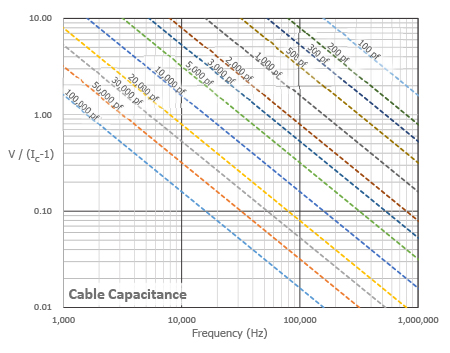# Driving Long Cables

Operation over long cables may affect frequency response and introduce noise and distortion when an insufficient current is available to drive cable capacitance.

Unlike charge output systems, where the system noise is a function of cable length, ICP® sensors provide a high voltage, low impedance output well-suited for driving long cables through harsh environments.

While there is virtually no increase in noise with ICP® sensors, the capacitive loading of the cable may distort or filter higher frequency signals depending on the supply current and the output impedance of the sensor.Figure 12: Long Extension Cable Schematic

Generally, this signal distortion is not a problem with lower frequency testing within a range up to 10,000 Hz. However, for higher frequency vibration, shock or transient testing over cables longer than 100 ft. (30 m.), the possibility of signal distortion exists.

The maximum frequency that can be transmitted over a given cable length is a function of both the cable capacitance and the ratio of the peak signal voltage to the current available from the signal conditioner according to:where, fmax= maximum frequency (hertz)
C= cable capacitance (picofarads)
V= maximum peak output from sensor (volts)
Ic= constant current from signal conditioner (mA)
109 = scaling factor to equate units

Note that in this equation, 1 mA is subtracted from the total current supplied to sensor (Ic). This is done to compensate for powering the internal electronics. Some specialty sensor electronics may consume more or less current. Contact the manufacturer to determine the correct supply current.

When driving long cables, the equation above shows that as the length of cable, peak voltage output or maximum frequency of interest increases, a greater constant current will be required to drive the signal.

The nomograph below provides a simple, graphical method for obtaining the expected maximum frequency capability of an ICP® measurement system. The maximum peak signal voltage amplitude, cable capacitance and supplied constant current must be known or presumed.For example, when running a 100 ft. (30 m) cable with a capacitance of 30 pF/ft (98 pF/m), the total capacitance is 3000 pF. This value can be found along the diagonal cable capacitance lines. Assuming the sensor operates at a maximum output range of 5 volts and the constant current signal conditioner is set at 2 mA, the ratio on the vertical axis can be calculated to equal 5. The intersection of the total cable capacitance and this ratio result in a maximum frequency of approximately 10.2 kHz.

The nomograph does not indicate whether the frequency amplitude response at a point is flat, rising or falling. For precautionary reasons, it is good general practice to increase the constant current (if possible) to the sensor (within its maximum limit) so that the frequency determined from the nomograph is approximately 1.5 to 2 times greater than the maximum frequency of interest.

Note that higher current levels will deplete battery-powered signal conditioners at a faster rate. Also, any current not used by the cable goes directly to power the internal electronics and will create heat. This may cause the sensor to exceed its maximum temperature specification. For this reason, do not supply excessive current over short cable runs or when testing at elevated temperatures.

To more accurately determine the effect of long cables, it is recommended to experimentally determine the high frequency electrical characteristics.

The method illustrated in Figure 13 involves connecting the output from a standard signal generator into a unity gain, low-output impedance (<5 ohm) instrumentation amplifier in series with the ICP® sensor. The extremely low output impedance is required to minimize the resistance change when the signal generator/amplifier is removed from the system.Figure 13: Long Cable Testing

In order to check system frequency/amplitude response, set the signal generator to supply the maximum amplitude of the expected measurement signal. Observe the ratio of the amplitude from the generator to that shown on the scope. If this ratio is 1:1, the system is adequate for your test. (If necessary, be certain to factor in any gain in the signal conditioner or scope.) If the output signal is rising (1:1.3 for example), add series resistance to attenuate the signal. Use of a variable 100 ohm resistor will help set the correct resistance more conveniently. Note that this is the only condition that requires the addition of resistance. If the signal is falling (1:0.75 for example), the constant current level must be increased or the cable capacitance reduced.

It may be necessary to physically install the cable during cable testing to reflect the actual conditions encountered during data acquisition. This will compensate for potential inductive cable effects that are partially a function of the geometry of the cable route.